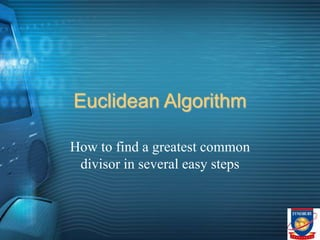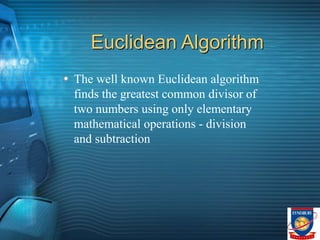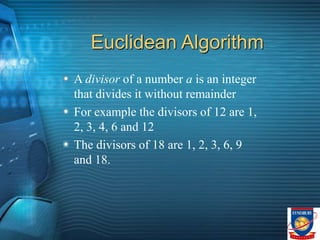Ce diaporama a bien été signalé.
Le téléchargement de votre SlideShare est en cours. ×
Publicité
Publicité
Publicité
Publicité
Publicité
Publicité
Publicité
Publicité
Publicité
Publicité
PublicitéChargement dans…3
×

## Consultez-les par la suite

1 sur 20 Publicité

# GCD.ppt

Mathematicians over the last two centuries have been used to the idea of considering a collection of objects/numbers as a single entity. These entities are what are typically called sets. The technique of using the concept of a set to answer questions is hardly new. It has been in use since ancient times. However, the rigorous treatment of sets happened only in the 19-th century due to the German mathematician Georg Cantor. He was solely responsible in ensuring that sets had a home in mathematics. Cantor developed the concept of the set during his study of the trigonometric series, which is now known as the limit point or the derived set operator. He developed two types of transfinite numbers, namely, transfinite ordinals and transfinite cardinals. His new and path-breaking ideas were not well received by his contemporaries. Further, from his definition of a set, a number of contradictions and paradoxes arose. One of the most famous paradoxes is the Russell’s Paradox, due to Bertrand Russell in 1918. This paradox amongst others, opened the stage for the development of axiomatic set theory. The interested reader may refer to Katz [

Mathematicians over the last two centuries have been used to the idea of considering a collection of objects/numbers as a single entity. These entities are what are typically called sets. The technique of using the concept of a set to answer questions is hardly new. It has been in use since ancient times. However, the rigorous treatment of sets happened only in the 19-th century due to the German mathematician Georg Cantor. He was solely responsible in ensuring that sets had a home in mathematics. Cantor developed the concept of the set during his study of the trigonometric series, which is now known as the limit point or the derived set operator. He developed two types of transfinite numbers, namely, transfinite ordinals and transfinite cardinals. His new and path-breaking ideas were not well received by his contemporaries. Further, from his definition of a set, a number of contradictions and paradoxes arose. One of the most famous paradoxes is the Russell’s Paradox, due to Bertrand Russell in 1918. This paradox amongst others, opened the stage for the development of axiomatic set theory. The interested reader may refer to Katz [

Publicité
Publicité

### GCD.ppt

1. 1. Euclidean Algorithm How to find a greatest common divisor in several easy steps
2. 2. Euclidean Algorithm The well known Euclidean algorithm finds the greatest common divisor of two numbers using only elementary mathematical operations - division and subtraction
3. 3. Euclidean Algorithm A divisor of a number a is an integer that divides it without remainder For example the divisors of 12 are 1, 2, 3, 4, 6 and 12 The divisors of 18 are 1, 2, 3, 6, 9 and 18.
4. 4. Euclidean Algorithm The greatest common divisor, or GCD, of two numbers is the largest divisor that is common to both of them. For example GCD(12, 18) is the largest of the divisors common to both 12 and 18.
5. 5. Euclidean Algorithm The common divisors of 12 and 18 are 1, 2, 3 and 6. Hence GCD(12, 18)=6.
6. 6. Euclidean Algorithm The Euclidean Algorithm to find GCD(a, b) relies upon replacing one of a or b with the remainder after division. Thus the numbers we seek the GCD of are steadily becoming smaller and smaller. We stop when one of them becomes 0.
7. 7. Euclidean Algorithm Specifically, we assume that a is larger than b. If b is larger than a, then we swap them around so that a becomes the old b and b becomes the old a. We then look for numbers q and r so that a=bq+r. They must have the properties that q0 and 0r<b. In other words, we seek the largest such q.
8. 8. Euclidean Algorithm As examples, consider the following. a=12, b=5; 12=5*2+2 so q=2, r=2 a=24, b=18; 24=18*1+6 so q=1, r=6 a=30, b=15; 30=15*2+0 so q=2, r=0 a=27, b=14; 27=14*1+13 so q=1, r=13 Try the ones on the next slide.
9. 9. Euclidean Algorithm Find q and r for the following sets of a and b. The answers are on the next slide. a=28, b=12 a=50, b=30 a=35, b=14 a=100, b=20
10. 10. Euclidean Algorithm Answers q=2, r=4 q=1, r=20 q=2, r=7 q=5, r=0
11. 11. Euclidean Algorithm The algorithm works in the following way. Given a and b, we find numbers q and r so that a=bq+r. We make sure that q is as large as possible (≥0), and 0≤r<b. For example, if a=18, b=12, then we write 18=12*1+6.
12. 12. Euclidean Algorithm Actually the number q isn’t important, it is just easier to find r with it when solving problems by hand. Most software can find the remainder r without finding q. For example the Java statement below will find r. r=a%b;
13. 13. Euclidean Algorithm Once the remainder r has been found we replace a by b and b by r. This relies on the fact that GCD(a,b)=GCD(b,r). Hence we repeatedly find r, the remainder after a is divided by b. Then replace a by b and b by r, and keep on in this way until r=0.
14. 14. Euclidean Algorithm Let us look at a graphical interpretation of the Euclidean algorithm. Obviously if p=GCD(a,b) then p|a and p|b, that is to say p divides both a and b evenly with no remainder.
15. 15. Euclidean Algorithm Suppose a and b are represented by the lengths below.
16. 16. Euclidean Algorithm Note that b does not go into a evenly, but has some small remainder.
17. 17. Euclidean Algorithm If p is the GCD of a and b then it divides evenly into both a and b. Hence it divides evenly into b and thus must divide evenly into both of the larger two boxes in the previous diagram.
18. 18. Euclidean Algorithm Then p divides the length representing b a whole number of times, and hence the boxes in a that represent whole lengths of b.
19. 19. Euclidean Algorithm Of course if p divides a evenly then it must also divide the remainder evenly. The picture below shows this.
20. 20. Euclidean Algorithm Hopefully it will be clear that by now any number that divides both a and b must also divide the remainder r. The largest of these will of course be the GCD of a and b. So GCD(a,b)=GCD(b,r).